## Example Questions

← Previous 1

### Example Question #1 : Quadratic Equations

x+ 3x + 2 = 0

What are the values of x that are solutions to the equation?

–1, –1

1, –2

–1, –2

–2, 0

0, 1

–1, –2

Explanation:

You factor the equation and get:

(x + 2)(x + 1) = 0

Then, you get x = –2 and = –1.

### Example Question #2 : Quadratic Equations

If x+ x – 6 = 0, what does x equal?

2, –3

–2, 3

0, –6

0, 6

6, 36

2, –3

Explanation:

There are two ways to solve this problem. One option is to use the quadratic formula: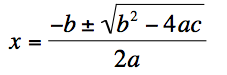In this problem, a = 1, b = 1, and c = –6. However, there is a lot of room for computational error using this method.

Another option is to factor the equation using the reverse FOIL method. Because we have x2, we know that both factors must include x. Thus, so far we have (x   )(x   ).  We also know that one factor must be negative and one must be positive if our last value (the L in FOIL) is to be negative. Thus, we have (x–  ) (x+ ). The factors of 6 are 1, 2, 3, and 6, so our last values must multiply together to make 6. Therefore, our options are (x – 1)(x + 6), (x – 6)(x + 1), (x – 2)(x + 3), and (x – 3)(x + 2). However, because we need our middle value to be 1, the combination of the outer and inner values (O and I in FOIL), must add up to positive 1. Since only a combination of +/–2 and +/–3 can add to 1, we can eliminate our first two options. Then, because we need the positive value to be larger than the negative value, we can determine that the correct factorization is (x – 2)(x + 3). When we set each of these pieces equal to zero, we get x – 2 = 0 and x + 3 = 0. Thus, X equals 2 and –3. We can check our work using FOIL.

### Example Question #3 : Quadratic Equations

Which of the following values ofare solutions to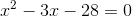?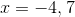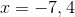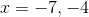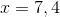Explanation:

1. Factor the above equation: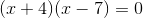2. Solve for: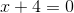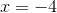and...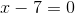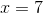### Example Question #8 : Quadratic Equations

Find the roots of the equation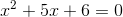.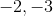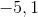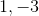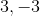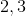Explanation:

To factor this, we need to find a pair of numbers that multiplies to 6 and sums to 5. The numbers 2 and 3 work. (2 * 3 = 6 and 2 + 3 = 5)

(x + 2)(x + 3) = 0

x = –2 or x = –3

### Example Question #2 : Quadratic Equations

In the equation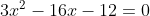what are the values of?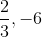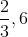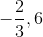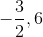Explanation:

To solve, begin by factoring the equation. We know that in our two factors, one will begin withand one will begin with. Fiddling with different factors of(we are looking for two numbers that, when multiplied byandseparately, will add to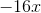), we come to the following: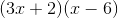(If you are unsure, double check by expanding the equation to match the original)

Now, set the each factor equal to 0: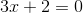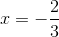Do the same for the second factor: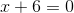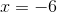Therefore, our two values ofare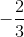and.

Alternatively, this problem can be solved by plugging each answer choice into the original equation and finding which set of numbers make the equation equate to 0.

### Example Question #1 : Quadratic Equations

Solve: x2+6x+9=0

12

6

9

3

-3

-3

Explanation:

Given a quadratic equation equal to zero you can factor the equation and set each factor equal to zero. To factor you have to find two numbers that multiply to make 9 and add to make 6. The number is 3. So the factored form of the problem is (x+3)(x+3)=0. This statement is true only when x+3=0. Solving for x gives x=-3. Since this problem is multiple choice, you could also plug the given answers into the equation to see which one works.

### Example Question #2 : Quadratic Equations

64x2 + 24x - 10 = 0

Solve for x

1/4 and -3/4
1/4 and 5/8
-1/4 and -5/8
1/4 and -5/8
-1/4 and 3/4
Explanation:

64x2 + 24x - 10 = 0

Factor the equation:

(8x + 5)(8x – 2) = 0

Set each side equal to zero

(8x + 5) = 0

x = -5/8

(8x – 2) = 0

x = 2/8 = 1/4

### Example Question #1 : Quadratic Equations

Which of the following is a root of the function?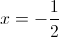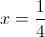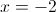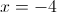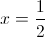Explanation:

The roots of a function are the x intercepts of the function. Whenever a function passes through a point on the x-axis, the value of the function is zero. In other words, to find the roots of a function, we must set the function equal to zero and solve for the possible values of x.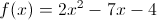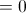This is a quadratic trinomial. Let's see if we can factor it. (We could use the quadratic formula, but it's easier to factor when we can.)

Because the coefficient in front of the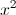is not equal to 1, we need to multiply this coefficient by the constant, which is –4. When we mutiply 2 and –4, we get –8. We must now think of two numbers that will multiply to give us –8, but will add to give us –7 (the coefficient in front of the x term). Those two numbers which multiply to give –8 and add to give –7 are –8 and 1. We will now rewrite –7x as –8x + x.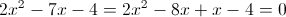We will then group the first two terms and the last two terms.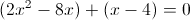We will next factor out a 2x from the first two terms.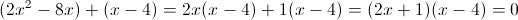Thus, when factored, the original equation becomes (2+ 1)(x – 4) = 0.

We now set each factor equal to zero and solve for x.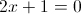Subtract 1 from both sides.

2x = –1

Divide both sides by 2.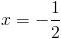Now, we set x – 4 equal to 0.

x – 4 = 0

x = 4

The roots of f(x) occur where x =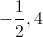.

The answer is therefore.

### Example Question #7 : Quadratic Equations

36x2 -12x - 15 = 0

Solve for x

-1/2 and 5/6

1/2 and 1/3

1/2 and -1/3

-1/2 and -5/6

1/2 and 5/6

-1/2 and 5/6

Explanation:

36x2 - 12x - 15 = 0

Factor the equation:

(6x + 3)(6x - 5) = 0

Set each side equal to zero

6x + 3 = 0

x = -3/6 = -1/2

6x – 5 = 0

x = 5/6

### Example Question #5 : Quadratic Equation

What is the sum of all the values ofthat satisfy: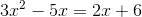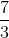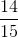Explanation:

With quadratic equations, always begin by getting it into standard form:Therefore, take our equation:And rewrite it as: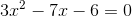You could use the quadratic formula to solve this problem.  However, it is possible to factor this if you are careful.  Factored, the equation can be rewritten as: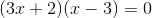Now, either one of the groups on the left could beand the whole equation would be.  Therefore, you set up each as a separate equation and solve for: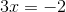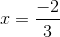OR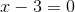The sum of these values is: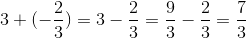← Previous 1